NCERT Solutions for Class 8 Maths Chapter 2 Linear Equations in One Variable Ex 2.2 are part of NCERT Solutions for Class 8 Maths. Here we have given NCERT Solutions for Class 8 Maths Chapter 2 Linear Equations in One Variable Ex 2.2.

 Board CBSE Textbook NCERT Class Class 8 Subject Maths Chapter Chapter 2 Chapter Name Linear Equations in One Variable Exercise Ex 2.2 Number of Questions Solved 16 Category NCERT Solutions

## NCERT Solutions for Class 8 Maths Chapter 2 Linear Equations in One Variable Ex 2.2

Question 1.
If you subtract $$\frac { 1 }{ 2 }$$ from a number and multiply the result $$\frac { 1 }{ 2 }$$ by you get $$\frac { 1 }{ 8 }$$ What is the number ?
Solution.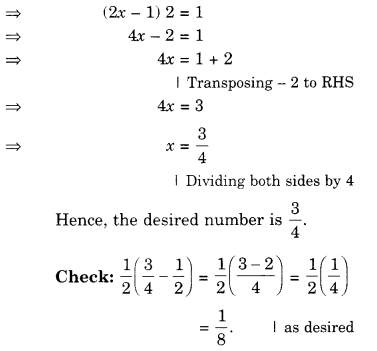Question 2.
The perimeter of a rectangular swimming pool is 154 m. Its length is 2 m more than twice its breadth. What are the length and the breadth of the pool?
Solution.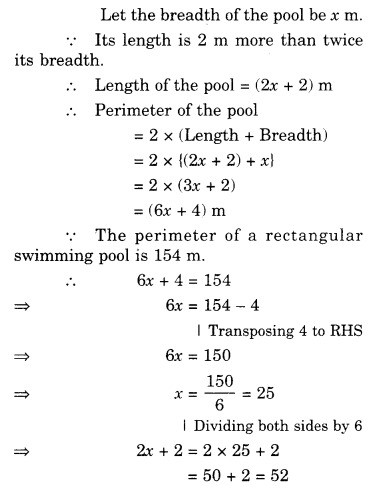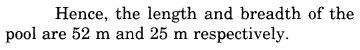Question 3.
The base of an isosceles triangle is $$\frac { 4 }{ 3 }$$cm. The perimeter of the triangle is $$4\frac { 2 }{ 15 }$$cm. What is the length of either of the remaining equal sides ?
Solution.Question 4.
Sum of two numbers is 95. If one exceeds the other by 15, find the numbers.
Solution.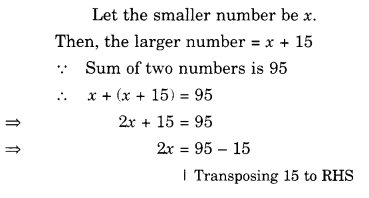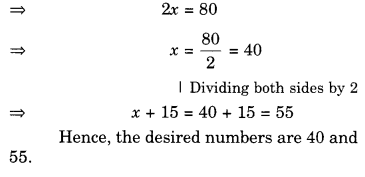Question 5.
Two numbers are in the ratio 5 :3. If they differ by 18, what are the numbers?
Solution.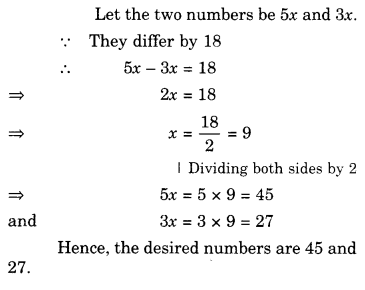Question 6.
Three consecutive integers add up to 51. What are these integers ?
Solution.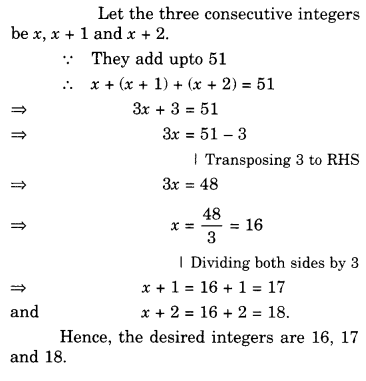Question 7.
The sum of three consecutive multiples of 8 is 888. Find the multiples.
Solution.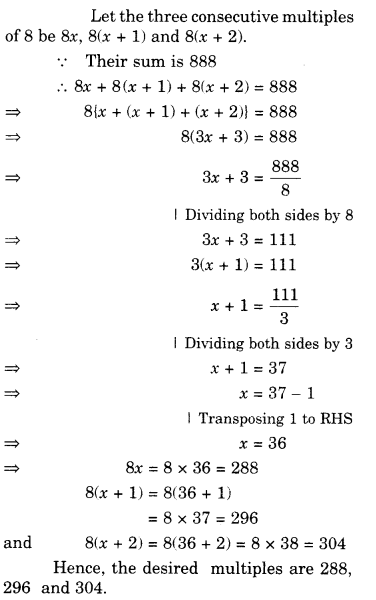Question 8.
Three consecutive integers are such that when they are taken in increasing order and multiplied by 2, 3 and 4 respectively, they add up to 74. Find these numbers.
Solution.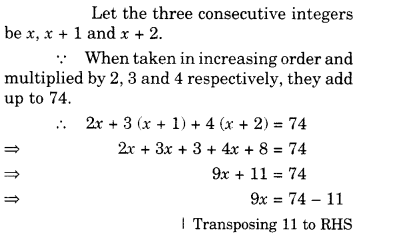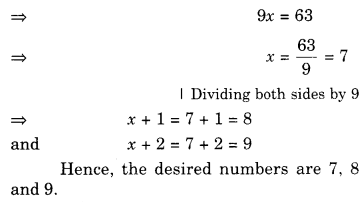Question 9.
The ages of Rahul and Haroon are in the ratio 5 : 7. Four years later, the sum of their ages will be 56 years. What are their present ages ?
Solution.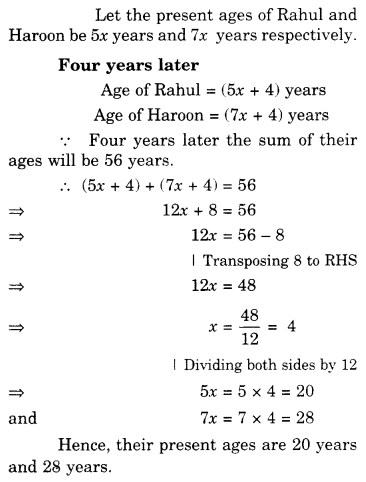Question 10.
The numbers of boys and girls in a class are in the ratio 7 : 5. The number of boys is 8 more than the number of girls. What is the total class strength ?
Solution..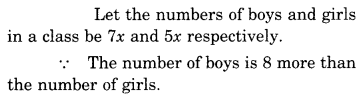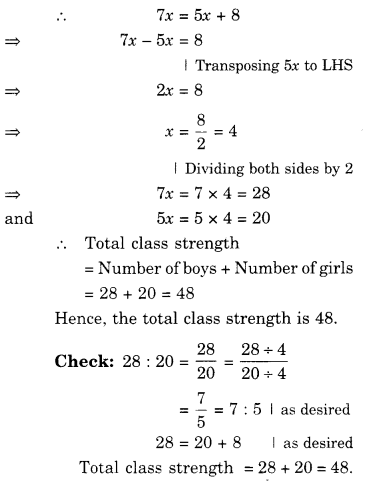Question 11.
Baichung’s father is 26 years younger than Baichung’s grandfather and 29 years older than Baichung. The sum of the ages of all the three is 135 years. What is the age of each one of them ?
Solution.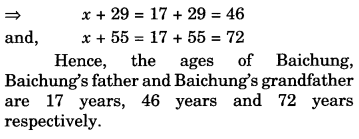Question 12.
Fifteen years from now Ravi’s age will be four times his present age. What is Ravi’s present age ?
Solution.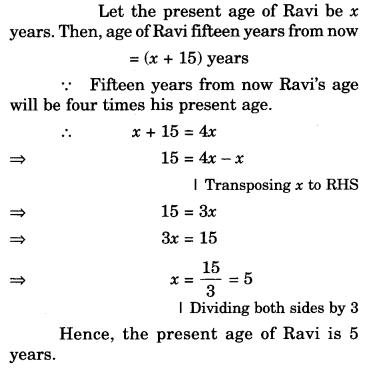Question 13.
A rational number is such that when you multiply it by $$\frac { 5 }{ 2 }$$ and add $$\frac { 2 }{ 3 }$$ to the product, you get$$-\frac { 7 }{ 12 }$$. What is the number ?
Solution.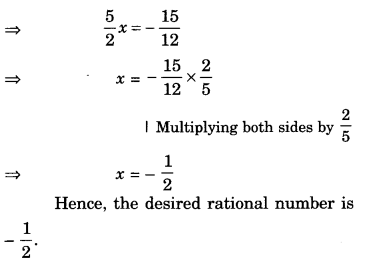Question 14.
Lakshmi is a cashier in a bank. She has currency notes of denominations ₹ 100, ₹ 50 and ₹ 10, respectively. The ratio of the number of these notes is 2 : 3 : 5. The total cash with Lakshmi is ₹ 4,00,000. How many notes of each denomination does she have ?
Solution.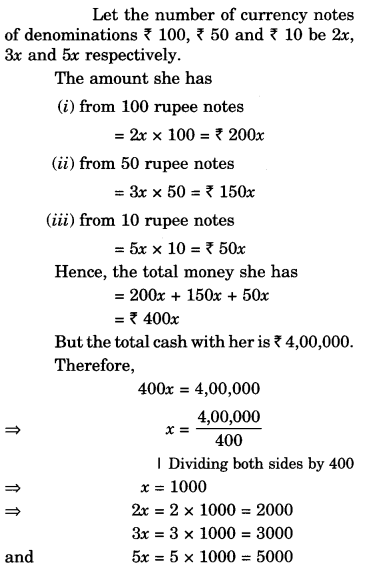Question 15.
I have a total of oft 300 in coins of denomination ₹ 1, ₹ 2 and ₹ 5. The number of ₹ 2 coins is 3 times the number of ₹ 5 coins. The total number of coins is 160. How many coins of each denomination are with me ?
Solution.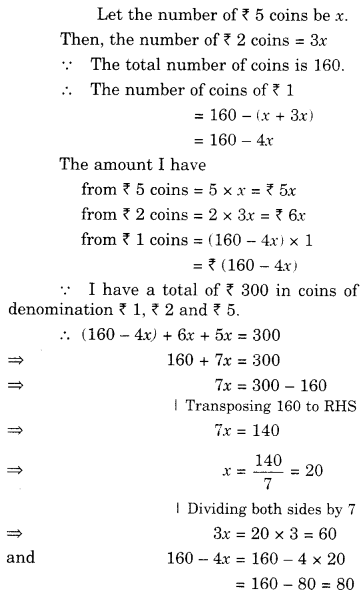Hence, I have 80, 60, and 20 coins of denomination ₹ 1, ₹ 2 and ₹ 5 respectively.

Question 16.
The organizers of an essay competition decide that a winner in the competition gets a prize of ₹ 100 and a participant who does not win gets a prize of ₹ 25. The total prize money distributed is ₹ 3,000. Find the number of winners, if the total number of participants is 63.
Solution.We hope the NCERT Solutions for Class 8 Maths Chapter 2 Linear Equations in One Variable Ex 2.2 help you. If you have any query regarding NCERT Solutions for Class 8 Maths Chapter 2 Linear Equations in One Variable Ex 2.2, drop a comment below and we will get back to you at the earliest.